qsort 订阅
qsort函数C语言编译器函数库自带的排序函数。qsort 的函数原型是void qsort(void*base,size_t num,size_t width,int(__cdecl*compare)(const void*,const void*)); 是base所指数组进行排序。qsort函数包含在C 标准库 - 中。  展开全文qsort函数C语言编译器函数库自带的排序函数。qsort 的函数原型是void qsort(void*base,size_t num,size_t width,int(__cdecl*compare)(const void*,const void*)); 是base所指数组进行排序。qsort函数包含在C 标准库 - 中。 

stdlib.h

qsort

C编程

qsort
qsort函数简介
void qsort(void *base, size_t nitems, size_t size, int (*compar)(const void *, const void*))回调函数：回调函数就是一个通过函数指针调用的函数。如果把函数的指针（地址）作为参数传递给另一个函数，当这个指针被用来调用其所指向的函数时，就说这是回调函数。   compar参数compar参数指向一个比较两个元素的函数。比较函数的原型应该像下面这样。注意两个形参必须是const void *型，同时在调用compar 函数（compar实质为函数指针，这里称它所指向的函数也为compar）时，传入的实参也必须转换成const void *型。在compar函数内部会将const void *型转换成实际类型。int compar(const void *p1, const void *p2);如果compar返回值小于0（< 0），那么p1所指向元素会被排在p2所指向元素的左面；　　如果compar返回值等于0（= 0），那么p1所指向元素与p2所指向元素的顺序不确定；　　如果compar返回值大于0（> 0），那么p1所指向元素会被排在p2所指向元素的右面。   使用排序例程进行排序。该函数不返回任何值。头文件：stdlib.h；

• qsort
2019-12-21 16:27:45

# 快速排序

## 代码实现

#include<iostream>
#include<algorithm>
using namespace std;

void qsort(int arr[], int left, int right) {
if(left < right) {
int i = left;
int j = right;
arr = arr[left];
while(i < j) {
while(i < j && arr <= arr[j]) j--;
arr[i] = arr[j];
while(i < j && arr >= arr[i]) i++;
arr[j] = arr[i];
}
arr[i] = arr;
qsort(arr, left, i-1);
qsort(arr, i+1, right);
}
}

void print(int arr[], int n) {
for(int i = 1; i <= n; i++) cout << arr[i] << " ";
cout << endl;
}

int main() {
int n;
cin >> n;
int a[n+1];
for(int i = 1; i <= n; i++) cin >> a[i];
qsort(a, 1, n);
print(a, n);
return 0;
}快速排序 算法 数据结构
更多相关内容
• 代码如下:/************************************************************************qsort原型：void qsort( void *base, size_t num, size_t width, int (__cdecl *compare )(const void *elem1, const void *...
• 这个方法是可以的，不用list的方法的话如果自己写一个qsort来做呢？ 在数组中找第n大的数，我知道输入数组长度是固定的，所以第n大就是第（数组长度 – n）小的数。然后对数组来排序，数组长度为length，设定两个位
• 本文实例为大家分享了C语言快排函数用法，供大家参考，具体内容如下 #include #include #include struct student { int id; char name;...int compare(const void* a,const void* b)//基本数据类型排序 ...
• 本文实例汇总了C语言中qsort函数的常见用法，非常具有实用价值。分享给大家供大家参考。具体分析如下： C语言中的qsort函数包含在的头文件里，本文中排序都是采用的从小到大排序。 一、对int类型数组排序 int num...
• //qsort的比较函数 int cmp(const void * a, const void * b) { return (*(Book*)a).num > (*(Book*)b).num ? 1 : 0; } //sort的比较函数 bool cmp_(Book a, Book b) { return a.num > b.num; } int main() { ...
• 快速排序算法在 Matlab 中的简单实现。 它是 O(N log N) 但唯一的优化是枢轴点是中心元素（以防止排序输入的病理行为）。 它需要一个 C 风格的比较函数。 如果要排序的向量是元胞数组，则比较函数对元胞内容起作用。...matlab
• 主要介绍了C语言中qsort函数的用法实例详解的相关资料,希望通过本文能帮助到大家，让大家理解掌握这部分内容，需要的朋友可以参考下
• 1.快排函数的介绍： 快速排序（Quicksort）是对冒泡排序的一种改进。也是最快的一种排序算法。 2.核心思想： 快速排序算法通过多次比较和交换来实现排序，其排序流程如下：  (1)首先设定一个分界值，通过该分界值将...
• c语言词典结构体匹配法，qsort与bsearchc语言
• qsort函数的简单使用这里写自定义目录标题1. 了解qsort函数2. 使用qsort函数对数组中的各种类型进行排序(1)整型元素排序(2)字符串中的字符元素排序(3)结构体元素排序3. 总结 大家好，我是十七，今天分享qsort函数的...

### qsort函数的简单使用

大家好，我是十七，今天分享qsort函数的简单使用

## 1. 了解qsort函数

(1) qsort是一个库函数，头文件是#include<stdlib.h>
(2) qsort是基于快速排序算法的排序函数

void qsort (void* base,
size_t num,
size_t size,
int (*compare)(const void* a,const void* b));
//1. base即为数组名，表示的是数组首元素的地址
//2. num表示的是数组中元素的个数
//3. size表示的数组中每个元素的字节大小
//4. a和b作为形参，接收的是数组中的两个不同元素

**//这里需要注意的是：compare是需要自己进行创建的一个函数**
**//然后把compare函数作为qsort的一个参数**
**//其中，整个compare就是基于快速排序逻辑的一个函数**


返回值如下：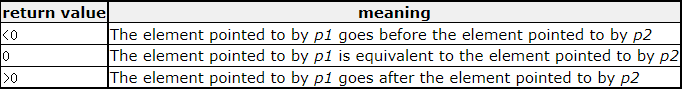## 2. 使用qsort函数对数组中的各种类型进行排序

直接上代码：

### (1)整型元素排序

#include <stdio.h>
#include <stdlib.h>
int compare(const void* a, const void* b);
int main(void)
{
int arr[] = { 9,7,8,4,5,6,1,2,3,0 };
int sz = sizeof(arr) / sizeof(arr);

qsort(arr,sz, sizeof(int), compare);

int i = 0;
for (i = 0; i < sz; i++)
{
printf("%d ", arr[i]);
}
printf("\n");
return 0;
}
int compare(const void* a, const void* b)
{
return (*(int*) a - *(int*) b);
}

//解释  qsort(arr,sz, sizeof(int), compare);
//1. arr是数组名，表示首元素的地址

//2. sz表示数组中所有元素的个数，这里sz = 10

//3. sizeof(int)是计算数组中的每个元素是多少字节的
//经计算后是4个字节，或者写成 sizeof(arr)

//4. compare表示创建一个函数
//规则就是接收两个无符号指针类型，目的在于排序任何类型的变量

//5. 解释  return (*(int*) a - *(int*) b);
//（1）将其先强制转换成int* 指针，目的是通过指针找到数组中的元素
//（2）再解引用，目的是比较每个元素的大小情况
//（3）最后返回


输出结果：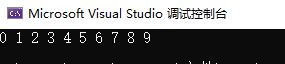注：如果我们把a和b调换位置，那么就会进行降序操作

#include <stdio.h>
#include <stdlib.h>
int compare(const void* a, const void* b);
int main(void)
{
int arr[] = { 9,7,8,4,5,6,1,2,3,0 };
int sz = sizeof(arr) / sizeof(arr);

qsort(arr,sz, sizeof(int), compare);

int i = 0;
for (i = 0; i < sz; i++)
{
printf("%d ", arr[i]);
}
printf("\n");
return 0;
}
int compare(const void* a, const void* b)
{
return (*(int*) b - *(int*) a);
}


输出结果：### (2)字符串中的字符元素排序

#include <stdio.h>
#include <string.h>
#include <stdlib.h>
int compare(const void* a, const void* b);
int main(void)
{
int len = strlen(arr);

qsort(arr, len, sizeof(char), compare);

printf("%s\n", arr);

return 0;
}
int compare(const void* a, const void* b)
{
return strcmp((char*)a, (char*)b);
//用strcmp比较字符串
}

//解释  qsort(arr, len, sizeof(char), compare);
//1. arr是数组名，表示首元素的地址

//2. len表示数组中待排序的字符的格式，不包括'\0'

//3. sizeof(char)是计算数组中的每个元素是多少字节的，经计算后是1个字节
//或者写成sizeof(arr)

//4. compare表示创建一个函数
//规则就是接收两个无符号指针类型，目的在于排序任何类型的变量

//5. 解释  return strcmp((char*)a, (char*)b);
//（1）将其先强制转换成char*指针，目的是通过指针找到数组中的元素
//（2）再解引用，目的是比较每个元素的大小情况
//（3）最后返回

//这里用到了strlen函数和strcmp函数，不再展开讲



注：如果我们把a和b调换位置，那么仍然会进行降序操作

### (3)结构体元素排序

1. 排序结构体中的字符
(1) 我们先创建一个 struct stu 类型的结构体，里面分别有名字、年龄、成绩 这三个变量
(2) 之后创建一个结构体数组，即用数组装了三个结构体变量
(3) 下面代码，目的是为了把人的名字进行排序，然后展示出来

这就好像我们大一在学校一样，用姓氏来排名
所以我创建了一个 comparebyname 函数进行排序

#include <stdio.h>
#include <string.h>
#include <stdlib.h>
int comparebyname(const void* a, const void* b);
struct stu
{
char name;
int age;
};
int main(void)
{
struct stu arr = { {"mingming",23,85}, {"yueyue", 20, 87},{"lili", 26, 90} };
int sz = sizeof(arr) / sizeof(arr);
int i = 0;
for (i = 0; i < sz; i++)
{
printf("%-15s %-10d %-10f\n", arr[i].name, arr[i].age, arr[i].grade);
}
printf("\n");

qsort(arr, sz, sizeof(struct stu), comparebyname);

for (i = 0; i < sz; i++)
{
printf("%-15s %-10d %-10f\n", arr[i].name, arr[i].age, arr[i].grade);
}
return 0;
}
int comparebyname(const void* a, const void* b)
{
return strcmp(((struct stu*)a)->name, ((struct stu*)b)->name);
}

//解释  qsort(arr, sz, sizeof(struct stu), comparebyname)
//1. arr是数组名，表示首元素的地址

//2. sz表示数组中所有元素的个数，这里sz = 3

//3. sizeof(struct stu)是计算数组中的每个元素是多少字节的,经计算后的值是28个字节
//(结构体内存对齐)
//这里的数组中每个元素是结构体变量，所以就是这么求得的,或者写成sizeof(arr)

//4. comparebyname表示创建一个函数，规则就是以字符串的首字符来进行排序整个数组

//5. return strcmp(((struct stu*)a)->name, ((struct stu*)b)->name);
//(1) (struct stu*)a 将无符号指针强制转换成一个结构体指针，目的是通过指针找到数组中的元素
//(2) ((struct stu*)a)->name 访问结构体成员变量
//(3) strcmp()接收的是两个地址，也就是指针类型
//(4) 这其中的name表示的是数组首元素的地址，所以符合strcmp要求


输出结果：
字符升序
l - m - y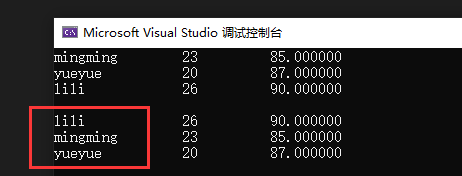同一个想法，不同逻辑
接着，我使用以年龄为排序方式

2. 排序结构体中的整型
同理，我创建了一个 comparebyage 函数

#include <stdio.h>
#include <string.h>
#include <stdlib.h>
int comparebyage(const void* a, const void* b);
struct stu
{
char name;
int age;
};
int main(void)
{
struct stu arr = { {"mingming",23,85}, {"yueyue", 20, 87},{"lili", 26, 90} };
int sz = sizeof(arr) / sizeof(arr);
int i = 0;
for (i = 0; i < sz; i++)
{
printf("%-15s %-10d %-10f\n", arr[i].name, arr[i].age, arr[i].grade);
}
printf("\n");

qsort(arr, sz, sizeof(struct stu), comparebyage);

for (i = 0; i < sz; i++)
{
printf("%-15s %-10d %-10f\n", arr[i].name, arr[i].age, arr[i].grade);
}
return 0;
}
int comparebyage(const void* a, const void* b)
{
return (*(struct stu*)a).age - (*(struct stu*)b).age;
}

//解释  qsort(arr, sz, sizeof(struct stu), comparebyage)
//1. arr是数组名，表示首元素的地址

//2. sz表示数组中所有元素的个数，这里sz = 3

//3. sizeof(struct stu)是计算数组中的每个元素是多少字节的,经计算后的值是 28个字节
//这里的数组中每个元素是结构体变量，所以就是这么求得的,或者写成sizeof(arr)

//4. comparebyage表示创建一个函数，规则就是以成绩的大小来进行排序整个数组

//5. return (*(struct stu*)a).age - (*(struct stu*)b).age;
//(1) (struct stu*)a 将无符号指针强制转换成一个结构体指针，目的是通过指针找到数组中的元素
//(2) (*(struct stu*)a).age 访问结构体成员变量
// age 是一个整型变量，所以必须解引用指针，然后访问的才是元素
//(3) 两个整型，在这qsort逻辑里面，直接用减号比较，然后返回就行了


输出结果：
数字升序
20 - 23 - 26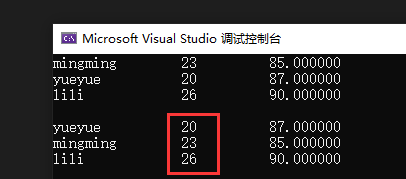## 总结

1. 本文意在表达如何正确、简单地使用qsort函数，并不打算介绍 qsort 函数内部算法的功能。qsort 函数是基于升序算法的，感兴趣的小伙伴可以模拟一下函数。
2. 本文没有讲到浮点类型的排序，因为它的逻辑和整形元素其实是一样的。
3. 本文用到了字符串函数，有如strlen、strcmp，这些并没有展开讲，不熟悉的小伙伴可以去MSDN，或者 cplusplus.com 去看一下，本文的 qsort函数 详细说明也可以看到介绍。
4. 本文也用到了数组、指针、结构体的一些知识，并将它们串了起来，对复习之前的C语言知识还是有亿点点帮助的，哈哈哈。

最后，本人是一名程序员小白，如果有大神看到了比较蹩脚、或是错误的代码，希望能指点一二，感谢！Over. 谢谢观看哟~

展开全文c语言
• ## C语言-qsort函数详解

千次阅读 多人点赞 2021-09-01 18:16:44
一.qsort函数是什么 二.使用qsort排序-以升序为例 关于void*型指针： 1.整形数组排序 2.字符数组排序 3.字符指针数组排序 4.结构体数组排序 5.浮点型数组排序 三.使用冒泡排序思想模拟实现qsort函数 1....

目录

一.qsort函数是什么

二.使用qsort排序-以升序为例

1.整形数组排序

2.字符数组排序

3.字符指针数组排序

4.结构体数组排序

5.浮点型数组排序

三.使用冒泡排序思想模拟实现qsort函数

1.什么是冒泡排序：

2.冒泡排序代码

3. 使用冒泡排序思想模拟实现qsort函数

## 一.qsort函数是什么

我们可以使用  搜索库函数网址或者MSDN软件进行查找。

qsort()函数：快速排序的函数  -引用stdlib.h头文件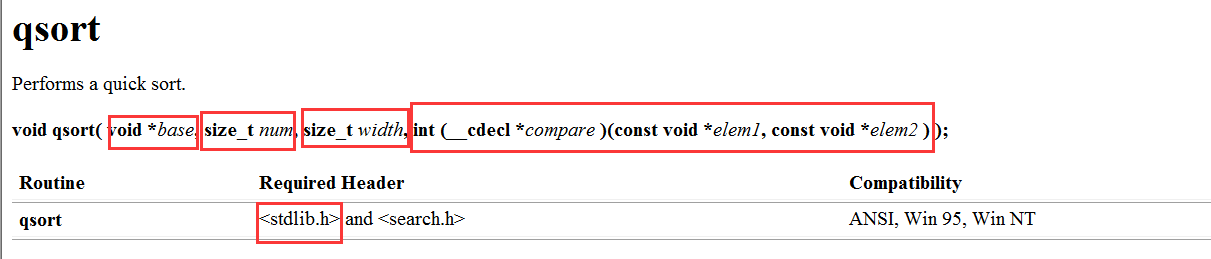参数说明：
void qsort (

void* base, //要排序的目标数组
size_t num,     //待排序的元素个数
size_t width,    //一个元素的大小，单位是字节
int(*cmp)(const void* e1, const void* e2)

);

其中cmp是函数指针，cmp指向的是：排序时，用来比较两个元素的函数。需要自己编写。

返回值：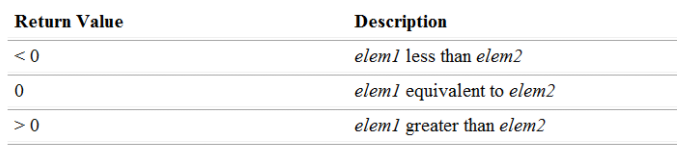## 二.使用qsort排序-以升序为例

### 关于void*型指针：

void*：无具体类型的指针   能够接收任意类型的地址
缺点：不能进行运算。不能+-整数，不能解引用

int a  = 0;
float f = 5.5f;
void* p1 = &a;
void* p2 = &f;
p1 = p1+1;    //err

## 1.整形数组排序

注意：

1.比较函数的参数类型为void* ，我们要进行强制类型转换！且要解引用才能得到对应的值！

2.若我们想排成降序，只需要写成e2-e1即可

void Print(int* arr, int sz)
{
int i = 0;
for (i = 0; i < sz; i++)
{
printf("%d ", *(arr + i));
}
printf("\n");
}
//比较整形
//注意类型时void* 所以要强制类型转化，还要解引用才是对应的值！！！
int cmp_int(const void* e1, const void* e2)
{
return *(int*)e1 - *(int*)e2;
}
void test1()
{
int arr[] = { 9,8,7,6,7,5,4,8 };
int sz = sizeof(arr) / sizeof(arr);
qsort(arr, sz, sizeof(arr), cmp_int);
Print(arr, sz);
}

## 2.字符数组排序

注意使用sizeof()操作符strlen()函数的区别

//注意要要强制类型转换！！ 要解引用！！！  本质上是比较Ascii值
int cmp_char(const void* e1, const void* e2)
{
return *(char*)e1 - *(char*)e2;
}
void test4()
{
char arr[] ="mango";
//若使用sizeof计算长度：
//int sz = sizeof(arr) / sizeof(arr);	//6
//qsort(arr, sz-1, sizeof(arr), cmp_float);
//因为sizeof把\0也算进去了，所以计算出来的值比字符串本身长度多1

int sz = strlen(arr);	//5
qsort(arr, sz, sizeof(arr), cmp_char);
printf("%s\n",arr);
}

## 3.字符指针数组排序

先看看下面这段程序有没有问题？

int cmp_chars(const void* e1, const void* e2)
{
return strcmp((char*)e1, *(char*)e2);
}
void test2()
{
char* arr1 = "abc";
char* arr3 = "cab";
char* p = { arr1,arr2,arr3 };
int sz = sizeof(p) / sizeof(p);
qsort(p, sz, sizeof(p), cmp_chars);
int i = 0;
for (i = 0; i < sz; i++)
{
printf("%s\n", p[i]);
}
}

打印出来发现：结果是错误的！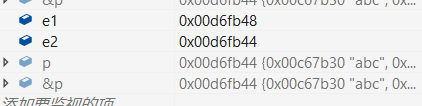->调试后发现：e2存放的是p的地址（char**类型），e1存放的是p指向的下一个元素的地址（char**类型）

对于这种写法，传进去的是p的地址，strcmp()会将p地址对应的内容转化成字符串，也就是将p中arr1，arr2，arr3的地址转化成字符串

实际上应该传p地址空间中arr1，arr2的地址，这样strcmp()才能找到arr1和arr2对应的字符串，因此得先把e1，e2转化成char**，这样解引用以后才是一个char*的地址

原因：把p传给qsort，p是数组名->首元素地址，元素类型为char*>，所以p的类型为：char**类型。  所以e1 和e2也要强制类型转化为char**，解引用e1，e2才是对应字符串的地址！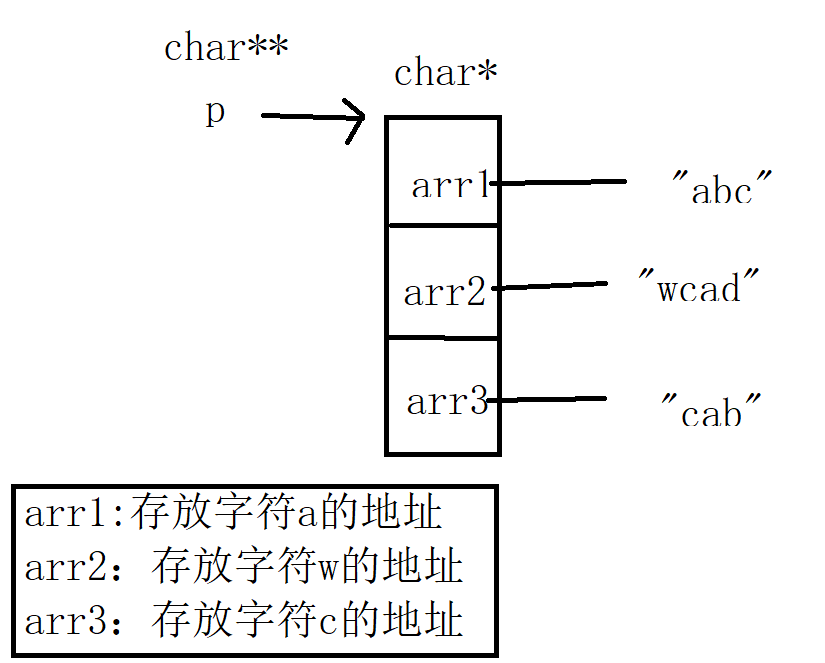正解：

int cmp_chars(const void* e1, const void* e2)
{
return strcmp(*(char**)e1, *(char**)e2);
}
void test2()
{
char* arr1 = "abc";
char* arr3 = "cab";
char* p = { arr1,arr2,arr3 };
int sz = sizeof(p) / sizeof(p);
qsort(p, sz, sizeof(p), cmp_chars);
int i = 0;
for (i = 0; i < sz; i++)
{
printf("%s\n", p[i]);
}

## 4.结构体数组排序

比较年龄->实际比较的是整形

比较名字->实际比较的是字符串->使用strcmp函数，不能使用 == 判断

struct Stu
{
int age;
char name;
};
//比较结构体中元素的年龄
int cmp_age(const void* e1, const void* e2)
{
//本质是比较整形
return ((struct Stu*)e1)->age - ((struct Stu*)e2)->age;
}
//比较名字
int cmp_name(const void* e1, const void* e2)
{
//本质是字符串比较->使用strcmp函数
return strcmp(((struct Stu*)e1)->name, ((struct Stu*)e2)->name);
}
void test2()
{
//创建结构体数组，用大括号初始化
struct Stu s = { {19,"Mango"},{18,"Lemon"},{20,"Hello"} };
int sz = sizeof(s) / sizeof(s);
//以年龄排
qsort(s, sz, sizeof(s), cmp_age);
printf("%s %d ",s.name,s.age);
printf("%s %d ", s.name, s.age);
printf("%s %d ", s.name, s.age);
printf("\n");
//以姓名排
qsort(s, sz, sizeof(s), cmp_name);
printf("%s %d ", s.name, s.age);
printf("%s %d ", s.name, s.age);
printf("%s %d ", s.name, s.age);
printf("\n");
}


## 5.浮点型数组排序

注意：比较函数中，返回类型是int，最后相减的值要强制类型转化为int ，但这也会造成错误，建议使用方法2.

//写法1：可能会出错
// 原因： 0.2 -0.1 = 0.1 强制类型转化为int后 结果为0
//int cmp_float(const void* e1, const void* e2)
//{
//	//返回类型是int  所以相减后的结果要强制类型转化
//	return (int)(*(float*)e1 - *(float*)e2);
//}

//写法2：对应上qsort的返回值
int cmp_float(const void* e1, const void* e2)
{
if ((*(float*)e1 - *(float*)e2) > 0.00000)
return 1;
else if ((*(float*)e1 - *(float*)e2) == 0.000000)
return 0;
else
return -1;
}
void test3()
{
float arr = { 5.01f,5.01f,0.02f,0.01f,5.001f };
int sz = sizeof(arr) / sizeof(arr);
qsort(arr, sz, sizeof(arr), cmp_float);
int i = 0;
for (i = 0; i < sz; i++)
{
printf("%f ", arr[i]);
}
}

## 三.使用冒泡排序思想模拟实现qsort函数

### 1.什么是冒泡排序：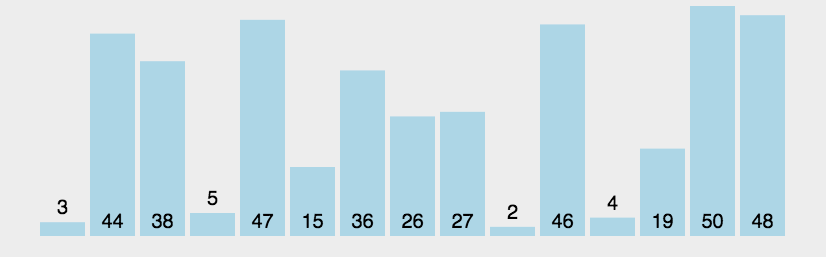主要思想：相邻的两个元素进行比较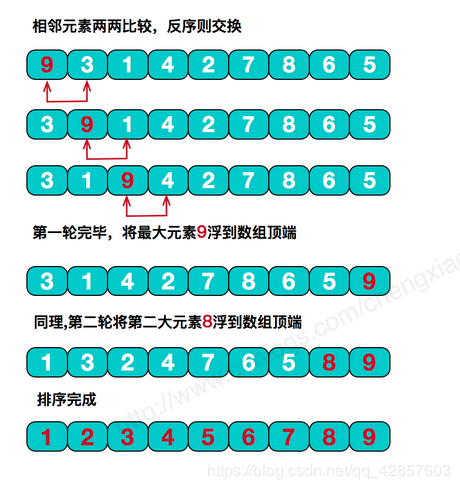对于冒泡排序： n个元素 共进行n-1趟冒泡排序。一趟可以使一个元素在特定位置上，每趟排序可以少比较一个元素

但是冒泡排序只能排序整形

### 2.冒泡排序代码

void BubbleSort(int* arr, int sz)
{
int i = 0;
int j = 0;
//共进行sz-1趟
for (i = 0; i < sz-1; i++)
{
int flag = 1;//每一趟进来都假设有序
// 每一趟
for (j = 0; j < sz - 1 - i; j++)
{
if (arr[j] > arr[j + 1])
{
int tmp = arr[j];
arr[j] = arr[j + 1];
arr[j + 1] = tmp;
flag = 0;
}
}
//若falg还是1，说明没有交换->已经有序了break退出
if (flag == 1)
{
break;
}
}
}
int main()
{
int arr = { 2,3,6,7,9,0,0,3,2,10 };
int sz = sizeof(arr) / sizeof(arr);
BubbleSort(arr, sz);
return 0;
}

### 3. 使用冒泡排序思想模拟实现qsort函数

qsort库函数使用的是什么参数，我们设计的函数就使用什么参数！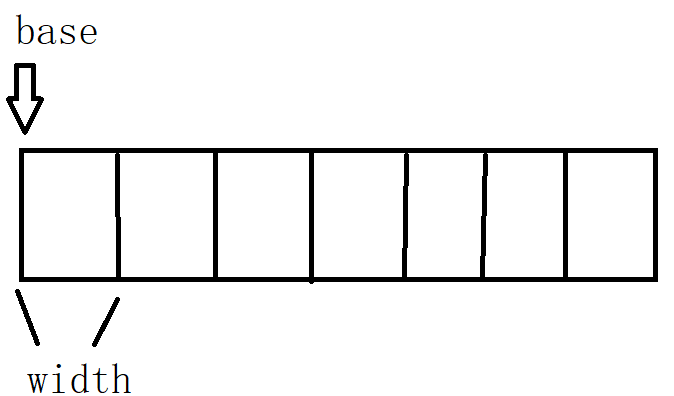1.为何将base强制类型转化为char*型指针：

原因：char* 指针+1跳过一个字节，+width：跳过width个字节，指向下一个元素。转化为其他类型不合适

2. 交换函数：还要把宽度（每个元素所占字节数）传过去
因为交换的时候是传地址，所以要知道元素的宽度，一个字节一个字节的交换 ，这样也证明了使用char*指针的好处！

3.(char*)base + j * width, (char*)base + (j + 1) * width,

当j = 0时：比较的是第一个元素和第二个元素
j = 1时，比较的是第二个元素和第三个元素
....  很妙的写法

//交换 --一个字节一个字节的交换，共交换width次
void Swap(char* buf1, char* buf2, size_t width)
{
size_t i = 0;
for (i = 0; i < width; i++)
{
char tmp = *buf1;
*buf1 = *buf2;
*buf2 = tmp;
buf1++;
buf2++;
}
}
void my_BubbleSort(void* base, size_t num,size_t width, int(*cmp)(const void* e1, const void* e2))
{
//冒泡排序
//若要排序n个元素，只需要进行n-1趟
//每一趟可以少比较一个元素，每一趟可以使一个元素在确定的位置上
//num：要排序元素的个数 类型是size_t
//num是无符号数 防止产生警告 所以i和j也定义为size_t
// size_t == unsigned int
size_t i = 0;
size_t j = 0;

//共进行num-1趟
for (i = 0; i < num; i++)
{
//每一趟
for (j = 0; j < num - 1 - i; j++)
{
//比较
//传地址
//相邻两个元素比较   width:宽度，每个元素所占字节
//排成升序
if (cmp((char*)base + j * width, (char*)base + (j + 1) * width) > 0)
{
//交换两数
Swap( (char*)base + j * width, (char*)base + (j + 1) * width, width );
}
}
}
}

当然 ，交换也可以使用库函数memcpy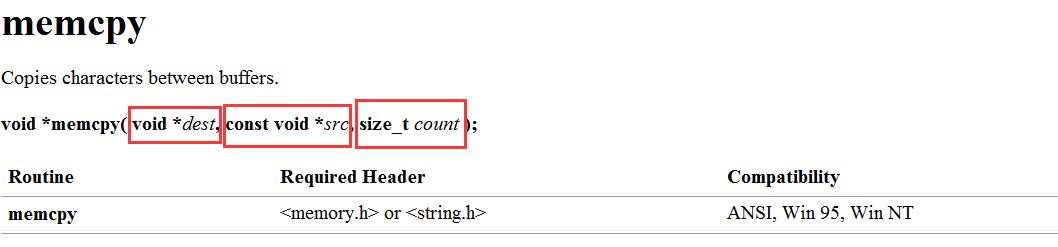dest：目标空间

src：要拷贝到目标空间的字符 -因为不作修改，所以可以用const修饰

count：字节数

char tmp ;    //防止结构体类型之类的类型    临时空间
memcpy(tmp, (char*)base + j * size, size);
memcpy( (char*)base + j * size,  (char*)base + (j + 1) * size, size);
memcpy( (char*)base + (j + 1) * size, tmp, size);


如果文章对你有帮助的，欢迎大佬们点个赞留言呀！如果有错误的话，请评论告知！

展开全文c语言 算法 排序算法 排序
• 文章目录qsort函数原型compar参数int 数组排序字符串数组排序字符串指针数组排序字符串二维数组排序整型二维数组 qsort函数原型 void qsort( void *base, size_t nmemb, size_t size, int (*compar)(const void ...

## 1. qsort函数原型

void qsort(
void *base,
size_t nmemb,
size_t size,
int (*compar)(const void *, const void *)
);


头文件:<stdlib.h>

函数功能：qsort()函数的功能是对数组进行排序，数组有nmemb个元素，每个元素大小为size。

参数base : base指向数组的起始地址，通常该位置传入的是一个数组名。

参数nmemb :nmemb表示该数组的元素个数

参数size :size表示该数组中每个元素的大小（字节数）

参数(*compar)(const void *, const void *):此为指向比较函数的函数指针，决定了排序的顺序。

函数返回值：无

注意：如果两个元素的值是相同的，那么它们的前后顺序是不确定的。也就是说qsort()是一个不稳定的排序算法。

## 2. compar参数

compar参数是qsort函数排序的核心内容，它指向一个比较两个元素的函数，注意两个形参必须是const void *型，同时在调用compar 函数（compar实质为函数指针，这里称它所指向的函数也为compar）时，传入的实参也必须转换成const void *型。在compar函数内部会将const void *型转换成实际类型，见下文。

int compar(const void *p1, const void *p2);


如果compar返回值小于0（< 0），那么p1所指向元素会被排在p2所指向元素的前面

如果compar返回值等于0（= 0），那么p1所指向元素与p2所指向元素的顺序不确定

如果compar返回值大于0（> 0），那么p1所指向元素会被排在p2所指向元素的后面

因此，如果想让qsort()进行从小到大（升序）排序，那么一个通用的compar函数可以写成这样：

//方法一
int compare (const void * a, const void * b)
{
if ( *(MyType*)a <  *(MyType*)b ) return -1;
if ( *(MyType*)a == *(MyType*)b ) return 0;
if ( *(MyType*)a >  *(MyType*)b ) return 1;
}


注意：你要将MyType换成实际数组元素的类型。

或者,如下所示。

//方法二
//升序排序
int compare (const void * a, const void * b)
{
return ( *(int*)a - *(int*)b );
}
//降序排列
int compare (const void * a, const void * b)
{
return ( *(int*)b - *(int*)a );
}


如果变量 a 指向一个较小的负整型数，b指向一个较大的正整型数，(*(int*)a - *(int*)b) 表达式会计算出一个正数，这是错误的。

因此，我们不能用减法来比较int的大小，这会产生溢出。取而代之地，我们可以使用大于、小于运算符来比较，如方法一所示。

## 3. int 数组排序

/* qsort example */
#include <stdio.h>
#include <stdlib.h>

int values[] = { 40, 10, 100, 90, 20, 25 };

int compare (const void * a, const void * b)
{
return ( *(int*)a - *(int*)b );
}

int main ()
{
int n;
qsort (values, sizeof(values)/sizeof(values), sizeof(int), compare);
for (n=0; n<sizeof(values)/sizeof(values); n++)
printf ("%d ",values[n]);
return 0;
}


版权声明：本文为博主原创文章，遵循 CC 4.0 BY-SA 版权协议，转载请附上原文出处链接和本声明。
本文链接：https://blog.csdn.net/qq_16933601/article/details/107214404

## 4. 结构体排序

#define _CRT_SECURE_NO_WARNINGS
#include <stdio.h>
#include<stdlib.h>
// void qsort(void* base, size_t num, size_t size, int(*compare)(const void*, const void*))

typedef struct
{
char name;   // 学生姓名
int Chinese;    // 语文成绩
int Math;        // 数学成绩
int English;     // 英语成绩
}st;
int cmp(const void* a, const void* b)
{
st* pa = (st*)a;
st* pb = (st*)b;
int num1 = pa->Chinese + pa->English + pa->Math;
int num2 = pb->Chinese + pb->English + pb->Math;

//return (int)num1 - num2;   // 从小到大，
return (int)num2 - num1;   //  从大到小
}
int main(void)
{
st students = {
{"周",97,68,45},
{"吴",100,32,88},
{"郑",78,88,78},
{"王",87,90,89},
{"赵",87,77,66},
{"钱",59,68,98},
{"孙",62,73,89}
};
qsort(students, 7, sizeof(st), cmp);   // 注意区别 students 与 st

for (int i = 0; i < 7; i++)
{
printf("%s%4d%4d%4d\t", students[i].name, students[i].Chinese, students[i].Math, students[i].English);
printf("总分：%d\n", students[i].Chinese + students[i].English + students[i].Math);
}

system("pause");
return 0;
}


## 5. 字符串指针数组排序

#include <stdio.h>
#include <string.h>
#include <stdlib.h>

int compare(const void *arg1, const void *arg2);

int
main(int argc, char** argv)
{
int i;

char *arr = { "i", "love", "c", "programming", "language" };

qsort(arr, sizeof(arr)/sizeof(arr), sizeof(char *), compare);

for (i = 0; i < 5; i++) {
printf("%s ", arr[i]);
}
printf("\n");

}

int compare(const void *arg1, const void *arg2) {
char *a = *(char**)arg1;
char *b = *(char**)arg2;
int result = strcmp(a, b);
if (result > 0) {
return 1;
}
else if (result < 0) {
return -1;
}
else {
return 0;
}
}


那么我们向qsort传入arr之后，qsort将arr理解为指向数组中第一个元素的指针，所以形参表中，arg1和arg2其实是指向“指向常量字符串的指针”的指针，是char**。而我们需要传给strcmp这个字符串比较函数的，是“指向字符串的指针”，是char*，所以我们将void*转换为char**，然后解引用，得到char*，赋予a和b。接下来使用strcmp对a和b进行比较。(数组名本身算一层指针，而里面的内容又是一层指针，数组存放的是指向字符串的地址)

## 6. 字符串二维数组排序

#include <stdio.h>
#include <string.h>
#include <stdlib.h>

int compare(const void *arg1, const void *arg2);

int
main(int argc, char** argv)
{
int i;

char arr = { "i", "love", "c", "programming", "language" };

qsort(arr, sizeof(arr)/sizeof(arr), sizeof(arr), compare);
printf("%s\n", arr);
for (i = 0; i < 5; i++) {
printf("%s ", arr[i]);
}
printf("\n");
}

int compare(const void *arg1, const void *arg2) {
char *a = (char*)arg1;
char *b = (char*)arg2;
int result = strcmp(a, b);
if (result > 0) {
return 1;
}
else if (result < 0) {
return -1;
}
else {
return 0;
}
}


这里对二维数组进行排序，其实是对二维数组的第二维中存放的字符串进行排序。所以qsort(arr, sizeof(arr)/sizeof(arr), sizeof(arr), compare);对qsort函数的调用中，第二个参数是待排元素的个数（5个），第三个参数是待排元素的大小（16）。

我们将arr传入qsort函数，qsort函数将arr理解为指向数组第一个元素的指针，arr的第一个元素是arr，所以参数arg1和arg2指的是指向"a[i]"的指针，我们知道，a[i]是字符，就是char，所以arg1和arg2指的是char *。我们将void*转换为char*，赋予a和b，调用strcmp函数对a和b进行比较。

## 7. qsort函数应用：整型二维数组

1. 最接近原点的 K 个点

我们有一个由平面上的点组成的列表 points。需要从中找出 K 个距离原点 (0, 0) 最近的点。（这里，平面上两点之间的距离是欧几里德距离。）你可以按任何顺序返回答案。除了点坐标的顺序之外，答案确保是唯一的。

示例 1：

输入：points = [[1,3],[-2,2]], K = 1
输出：[[-2,2]]
解释： (1, 3) 和原点之间的距离为sqrt(10)， (-2, 2) 和原点之间的距离为 sqrt(8)， 由于 sqrt(8) < sqrt(10)，(-2, 2) 离原点更近。 我们只需要距离原点最近的 K = 1 个点，所以答案就是 [[-2,2]]。
示例 2：
输入：points = [[3,3],[5,-1],[-2,4]], K = 2
输出：[[3,3],[-2,4]] （答案 [[-2,4],[3,3]] 也会被接受。）
提示：
1 <= K <= points.length <= 10000
-10000 < points[i] < 10000
-10000 < points[i] < 10000

/* qsort排序二维数组，cmp的每个元素都是一个独立的 int 数组，也就是指针 */
int cmp(const void* a, const void* b) {

// 转换为对应一维数组
int* arry1 = *(int**)a;
int* arry2 = *(int**)b;

// 获取对应arry1 的两个元素
int x1 = *arry1;
int y1 = *(arry1 + 1);

int x2 = *arry2;
int y2 = *(arry2+1);

return (x1*x1 + y1*y1) - (x2*x2 + y2*y2);
}

int** kClosest(int** points, int pointsSize, int* pointsColSize, int K, int* returnSize, int** returnColumnSizes){
if(points==NULL || pointsSize==0 || K==0) return NULL;

qsort(points, pointsSize, sizeof(int)*pointsColSize, cmp);
/*   这里注意 qsort 的传参，使用不当会报错
Line 11: Char 11: runtime error: load of misaligned address 0x602000000032 for type 'int *', which requires 8 byte alignment (solution.c)       0x602000000032: note: pointer points here
*/

// 指定return输出的二维数组是包含有几个一维数组
*returnSize = pointsSize > K ? K : pointsSize;

*returnColumnSizes = (int*)malloc(sizeof(int*)*(*returnSize));
// 指定每个一维数组的 col
for(int i = 0; i< (*returnSize); i++) {
(*returnColumnSizes)[i] = 2;
}
return points;
}



养成习惯，先赞后看！如果觉得写的不错，欢迎关注，点赞，在看，转发，谢谢！展开全文C语言 数据结构与算法 排序
• ## qsort库函数详解

千次阅读 2022-03-14 16:39:41
一、qsort是什么？ 二、qsort的功能? 三、qosrt函数详解 1.qsort函数的定义 2.qsort函数的传参 四、qsort的模拟实现(冒泡) 1.如何实现交换？ 2.如何实现字节之间的交换 一、qsort是什么？ qsort是C语言...c语言 c++ 开发语言
• 本篇文章我们来了解一下回调函数以及C语言中qsort函数的使用方法。 C语言中qosrt函数的使用 ...c语言 后端 c++
• qsort的详细用法，可以用于自学。容易入门，懂
• qsort的函数原型： void qsort (void* base,size_t num,size_t size,int (* compar) (const void*e1,const void*e2) 现在对qsort函数原型进行分析： 1.void qsort： 这个void代表qsort函数是一个无返回类型的函数。...排序算法 数据结构 算法
• C库函数qsort的实现，对学习指针有极大的帮助。可以实现任意类型数据的排序。
• ## qsort函数用法详解

千次阅读 多人点赞 2020-12-03 21:01:14
qsort函数原理与应用详解 limabean 本文版权归作者所有,欢迎转载,但未经作者同意必须在文章页面给出原文链接,否则保留追究法律责任的权利。 1.qsort函数简介 排序是程序员经常碰到的问题，数据结构与算法的教科书上...c++
• 近来其实常用到qsort库函数。用法很简单qsort 的函数原型是void __cdecl qsort ( void *base, size_t num, size_t width, int (__cdecl *comp)(const void *, const void* ) )其中base是排序的一个集合数组，num是这...
• 文章目录一、qsort函数简介二、qsort函数常用实例1.比较整型数组2.比较char数组3.比较double数组4.比较字符串4.1按首字母排序4.2按长度排序4,3按字典顺序5.结构体排序5.1 多级排序三、qsort深度剖析 一、qsort函数...c语言 排序算法 算法
• ## 用qsort函数实现快速排序

千次阅读 多人点赞 2022-02-03 11:24:20算法
• 排序之qsort函数qsort函数是C语言库提供的快速排序的库函数，位于头文件在一般应用的时候我们应该尽量选择使用库函数，因为库函数一般都是经过优化总结出来的C代码，这样也可以少写大篇幅的代码。1.qsort函数之int型...
• qsort函数的作用是将所有数据排序，那么它和普通的冒泡排序或者选择排序有什么区别呢？它不仅仅可以排序数组中的数字，还可以排序结构体。当然升序和降序它都支持，不过输入参数的顺序会有所不同，下面我们来详细...排序算法 算法 c语言 c++ 数据结构
• qsort 函数是基于快速排序算法实现的一个排序函数 函数基本原型 void qsort( void *base, size_t num, size_t width, int (*cmp)(const void *elem1, const void *elem2 ) //函数指针 ); 参数解...c语言...